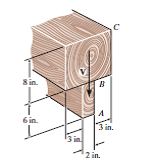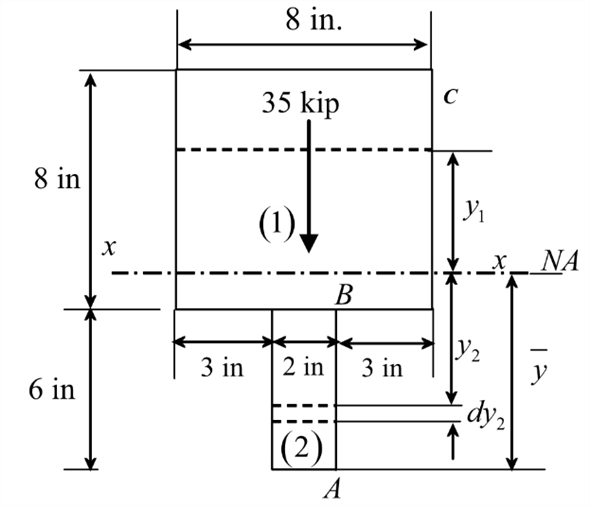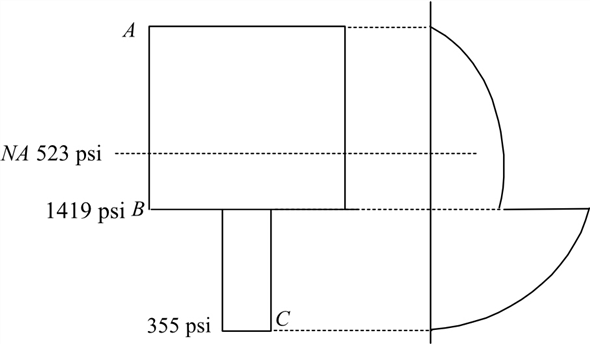Problem

# Sketch the intensity of the shear-stress distribution acting over the beam’s cross-secti...

Sketch the intensity of the shear-stress distribution acting over the beam’s cross-sectional area, and determine the resultant shear force acting on the segment AB . The shear acting at the section is V = 35 kip . Show that INA = 872.49 in4.#### Step-by-Step Solution

Solution 1

Sketch the cross section of the beam.Consider $$\bar{y}$$ as the distance of the neutral axis $$x-x$$ of the section from the bottom. Calculate the centroid for the cross section at neutral axis using the following equation:

$$\bar{y}=\frac{A_{1} y_{1}+A_{2} y_{2}}{A_{1}+A_{2}}$$

Here, $$A_{1}$$ and $$A_{2}$$ are the areas of the sections $$(1)$$ and $$(2)$$ and $$y_{2}, y_{1}$$ is the distance of the centroids for the sections (1) and (2).

Calculate the value $$y_{1}$$ as follows:

\begin{aligned} y_{1} &=\left(6+\frac{8}{2}\right) \\ &=10 \mathrm{in} \end{aligned}

Calculate the value $$y_{2}$$ as follows:

\begin{aligned} y_{2} &=\frac{6}{2} \\ &=3 \mathrm{in} . \end{aligned}

Substitute $$(8 \times 8)$$ in. $$^{2}$$ for $$A_{1}, 10$$ in for $$y_{1},(6 \times 2)$$ in. $$^{2}$$ for $$A_{2}$$, and 3 in. for $$y_{2}$$.

\begin{aligned} \bar{y} &=\frac{(8 \times 8) \times 10+(6 \times 2) \times 3}{(8 \times 8)+(6 \times 2)} \\ &=8.895 \mathrm{in} . \end{aligned}

Determine moment of inertia $$I_{N 4}$$ of the section about the neutral axis by apply the parallel axis theorem.

\begin{aligned} I_{\mathrm{MA}} &=\left(I_{1}+A_{1} d_{\mathrm{x}}^{2}\right)+\left(I_{2}+A_{2} d_{2}^{2}\right) \\ &=\left[\frac{\left(b_{1}\right) \times\left(h_{\mathrm{t}}\right)^{3}}{12}+\left(A_{1}\right) \times\left(y_{1}-\bar{y}\right)^{2}+\frac{\left(b_{2}\right) \times\left(h_{2}\right)^{3}}{12}+\left(A_{2}\right) \times\left(\bar{y}-y_{2}\right)^{2}\right] \end{aligned}

Here, $$b_{1}$$ is the width of the section (1), $$h_{1}$$ is the height of the section (1), $$b_{2}$$ is the width of the section (1), $$h_{2}$$ is the height of the section $$(2), d_{1}$$ and $$d_{2}$$ are the distance of the centroid from the to the neutral axis to that particular section, $$\bar{y}$$ is the distance of the neutral axis $$x-x$$ of the section from the bottom, and $$y_{2}, y_{1}$$ are the distance of the centroids for the sections (1) and (2).

Substitute 8 in. for $$b_{1}, 2$$ in. for $$b_{2}, 4$$ in. for $$h_{1}$$, and 2 in. for $$h_{2},(10-8.895)$$ in. for $$d_{1}$$, and $$(2.667-2)$$ in. for $$d_{2}$$

\begin{aligned} I_{\text {MA }} &=\frac{(8) \times(8)^{3}}{12}+(8 \times 8) \times(10-8.895)^{2}+\frac{(2) \times(6)^{3}}{12}+(2 \times 6) \times(8.895-3)^{2} \\ &=872.49 \text { in. }^{4} \end{aligned}

Compute the shear stress in portion $$B C$$ :

Calculate the moment of area about neutral axis.

$$Q_{1}=A_{1} \times y_{1}$$

Here, $$A_{1}$$ is the area of cross section above $$y_{1}$$ attached to the beam and $$y_{1}$$ is the distance of the centroid of section (1) from the neutral axis.

Substitute $$\left[\left(8+6-8.895-y_{1}\right) \times(8)\right]$$ in. $$^{2}$$ for $$A_{1}$$ and $$\left(y_{1}+\frac{8+6-8.895-y_{1}}{2}\right)$$ for $$y_{1}$$

\begin{aligned} Q_{1} &=\left(8+6-8.895-y_{1}\right) \times(8) \times\left(y_{1}+\frac{8+6-8.895-y_{1}}{2}\right) \\ &=4 \times\left(26.061-y_{1}^{2}\right) \mathrm{in}^{3} \end{aligned}

Calculate the shear stress at a distance $$y_{1}$$ from neutral axis using the following relation:

$$\tau_{1}=\frac{V Q_{1}}{I_{N A} t_{1}}$$

Here, $$V$$ is the shear stress, $$Q_{1}$$ is the moment of area $$A_{1}$$ about neutral axis, $$I_{N A}$$ is the moment of inertial about neutral axis, and $$t_{1}$$ is the thickness of the section at $$y_{1}$$.

Substitute $$35 \times 10^{3} \mathrm{lb}$$ for $$V, 4 \times\left(26.061-y_{1}^{2}\right)$$ in. $${ }^{3}$$ for $$Q_{1}, 872.49 \mathrm{in} .{ }^{4}$$ for $$I_{N A}$$ and 8 in. for $$t_{1}$$.

\begin{aligned} \tau_{1} &=\frac{\left(35 \times 10^{3}\right) \times 4 \times\left(26.061-y_{1}^{2}\right)}{(872.49) \times(8)} \ldots \ldots .(1) \\ &=20.0575 \times\left(26.061-y_{1}^{2}\right) \mathrm{psi} \end{aligned}

Consider the portion $$A B$$.

Determine moment of area about neutral axis.

$$Q_{2}=A_{2} \times y_{2}$$

Here, $$A_{2}$$ is the area of cross section below $$y_{2}$$ attached to the beam, and $$y_{2}$$ is the distance of the centroid of the section from the neutral axis.

Substitute $$\left[\left(8.895\right.\right.$$ in $$\left.-y_{2}\right) \times(2$$ in $$\left.)\right]$$ for $$A_{2}$$ and $$\left(y_{2}+\frac{8.895 \text { in }-y_{2}}{2}\right)$$ for $$y_{2}$$.

\begin{aligned} Q_{2} &=\left(8.895 \text { in }-y_{2}\right) \times(2 \text { in }) \times\left(y_{2}+\frac{8.895 \text { in }-y_{2}}{2}\right) \\ &=\left(79.121-y_{2}^{2}\right) \text { in }^{3} \end{aligned}

Calculate the shear stress $$\tau_{2}$$ at a distance $$y_{2}$$ from neutral axis using the following relation:

$$\tau_{2}=\frac{V Q_{2}}{I_{M A} t_{2}}$$

Here, $$V$$ is the shear stress, $$Q_{2}$$ is the moment of area $$A_{1}$$ about neutral axis, $$I_{N A}$$ is the moment of inertial about neutral axis, and $$t_{2}$$ is the thickness of the section at $$y_{1}$$.

Substitute $$35 \times 10^{3} \mathrm{lb}$$ for $$V,\left(79.121-y_{2}^{2}\right)$$ in. $$^{3}$$ for $$Q_{2}, 872.49$$ in $$^{4}$$ for $$I_{N A}$$ and 2 in. for $$t_{2}$$ in the equation for $$\tau_{2}$$.

\begin{aligned} \tau_{2} &=\frac{\left(35 \times 10^{3}\right) \times\left(79.121-y_{2}^{2}\right)}{(872.49) \times(2)} \\ &=20.0575 \times\left(79.121-y_{2}^{2}\right) \mathrm{psi} \end{aligned}

Draw the sketch of the section and show the shear stress distribution acting over the beam’s cross sectional area.Consider an element of thickness $$d y_{2}$$ at a depth $$y_{2}$$ from neutral axis.

Calculate the shear force over the section $$A B$$.

$$V_{A B}=\int_{y-6}^{y} \tau_{2} \times 2 d y_{2}$$

Here, thickness of the element is $$d y_{2}$$ and $$\tau_{2}$$ is the shear stress at a distance $$y_{2}$$ from neutral axis.

Substitute $$20.0575 \times\left(79.121-y_{2}^{2}\right)$$ psi for $$\tau_{2}$$ and $$8.895$$ in. for $$\bar{y}$$.

\begin{aligned} V_{A B} &=\int_{8.895-6}^{8.899} 20.0575 \times\left(79.121-y_{2}^{2}\right) \times 2 \times d y_{2} \\ &=20.0575 \times 2\left[79.121 y_{2}-\frac{y_{2}^{3}}{3}\right]_{8.895-6}^{8.895} \\ &=40.115 \times\left[79.121(6)-\left(\frac{679.51845}{3}\right)\right] \\ &=9957.33 \mathrm{ps} \\ &=9.96 \mathrm{kips} \end{aligned}

Therefore, the resultant shear force acting on the segment $$A B$$ is $$9.96 \mathrm{kips}$$.